Area between Polar Curves

• Last Updated : 14 Jul, 2021

Coordinate systems allow the mathematical formulation of the position and behavior of a body in space. These systems are used almost everywhere in real life. Usually, the rectangular Cartesian coordinate system is seen, but there is another type of coordinate system which is useful for certain kinds of curves. This coordinate system is the polar coordinate system. The polar coordinate system works on the basis of an angle and the distance from the origin. These coordinate systems are very useful in the case of shapes like spirals, circles, etc. It becomes essential to study how to find the area under the curves using this coordinate system. Let’s look at it in detail.

Polar Coordinate System

Coordinate systems are nothing but a way to define the position of a point in space. A rectangular or Cartesian coordinate system uses the horizontal(x) and vertical distance(y) of a point from the origin. Up until now, the rectangular coordinate system has been the focus of every mathematical concept. But it’s not always easy to deal with rectangular coordinates. The position of a point can also be described using the distance(r) of that point from the origin and the angle(θ) it makes with the x-axis. The figure below shows the position of a point with respect to the origin of both of these coordinate systems.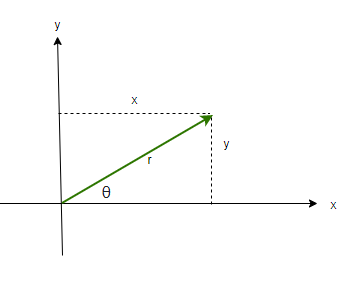These coordinates (r, θ) are called polar coordinates. Many curves are easily described in this manner. For example, the equation of the circle in the Cartesian system is given by,

x2 + y2 = a2

The equation of the same curve is polar coordinates will be given by,

r = a

This means that the curve includes all the points on the plane whose distance from the origin is “a”. Notice, that there is no θ in the equation, which means that whatever the angle made by the point from the x-axis. The distance is independent of that angle and is given by “a”.

Area defined by Polar Graphs

Polar graphs are the curves that are defined in the polar coordinate systems. The graphs of the equations in the polar coordinate systems are called polar graphs. The concept of the area under the curve is the same for both cases – the rectangular coordinate system and the polar coordinate system. The figure below represents a polar graph and region “A” denotes the region between θ = a and θ = b.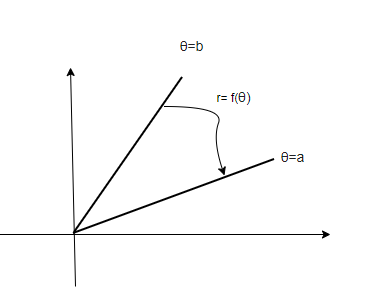Notice that in this region, the angle is changing slowly. This region can be divided into very small sectors as shown in the figure below. The sum of the area of these sectors will be the area enclosed by the curve.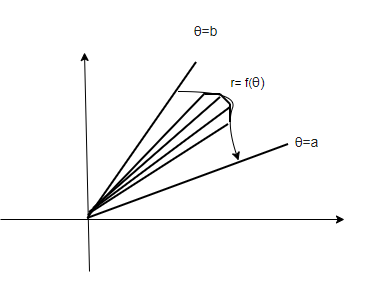To find the total area, consider one sector. The area of a sector in a circle is given by,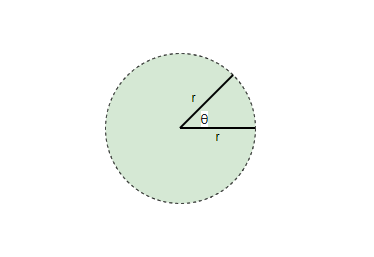Now, since the angle is very small, it can be written as dθ. Then, the area of a small sector becomes,The total area of the region is given by the sum of all such rectangles,This can be re-written as,Area enclosed by a Cardioid

A cardioid is a polar curve that resembles the shape of a heart. The shape of the cardioid can also be compared to the shape of an apple. It is a curve that is defined as the locus of a point lying on the circumference of a circle that is rolling without any slip. The graph and the equation of the curve is given below,

r = 1 + cos(θ)

or

r= 1 – cos(θ)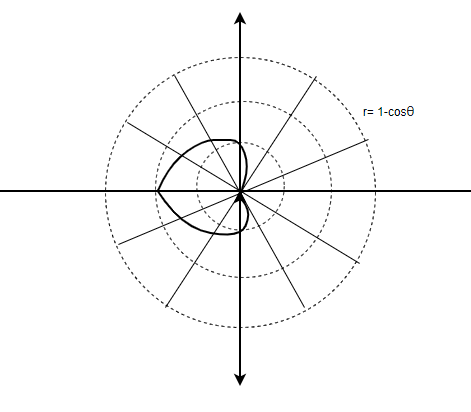Question: Find the area under this curve from θ = 0 to θ = 90°.In this case, a = 0 and b = 90° and r = 1 – cos(θ)

Plugging these values into the formula,Rearranging the equation with the identity cos(2θ) = 1 – 2cos2(θ)

A =A =A =Area Between two Polar Curves

All the concepts and the methods that apply for calculating different areas in Cartesian systems can be easily extended to the polar graphs. Consider two polar graphs that are given by, r = 3sin(θ) and r = 3cos(θ). The goal is to calculate the area enclosed between these curves. The graphs of these curves are given below;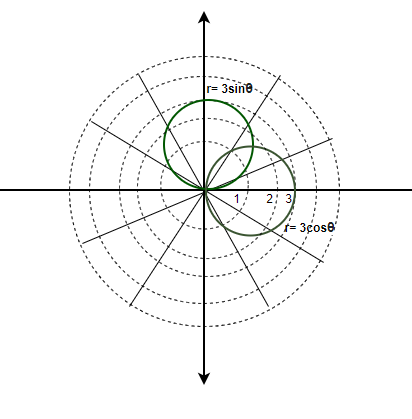The common region can be divided into two equal parts by the line passing through as shown in the figure below. The area of the intersection will be,

A =The total area will be twice the calculated area.

Sample Problems

Question 1: Find the area enclosed by the curve r = 3 in the first quadrant.

The given equation is the equation of a circle. The area can be calculated using the formula studied above,

A =A =A =A =Question 2: Find the area enclosed by the curve r = 5 + θ in the first two quadrants.

The given equation is the equation of a circle. The area can be calculated using the formula studied above,

A =A =A =A =A =Question 3: Find the area enclosed by the curve r = 5 + eθ in the first two quadrants.

The given equation is the equation of a circle. The area can be calculated using the formula studied above,

A =A =A =A =A =Question 4: Find the area under this curve from θ = 0 to θ = 180°.

r = 1 + cos(θ)In this case, a = 0 and b = 180° and r = 1 + cos(θ)

Plugging these values into the formula,Rearranging the equation with the identity cos(2θ) = 1 – 2cos2(θ)

A =A =A =A =Question 5: Find the area outside the circle r = 3 and inside the circle r = 5.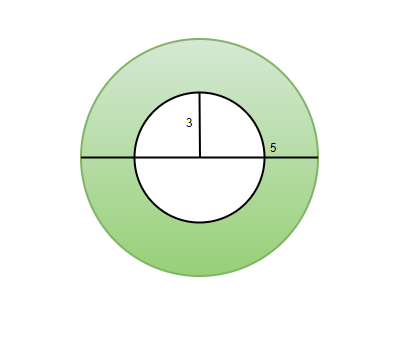The given equation is the equation of a circle. The area can be calculated using the formula studied above,

Area of the bigger circle,

A5A5A5A5The area of smaller circle will be,

A3A3A3A3The area of the common region will be,

A = A5 – A3

A = 50π – 9π

A = 41π

My Personal Notes arrow_drop_up